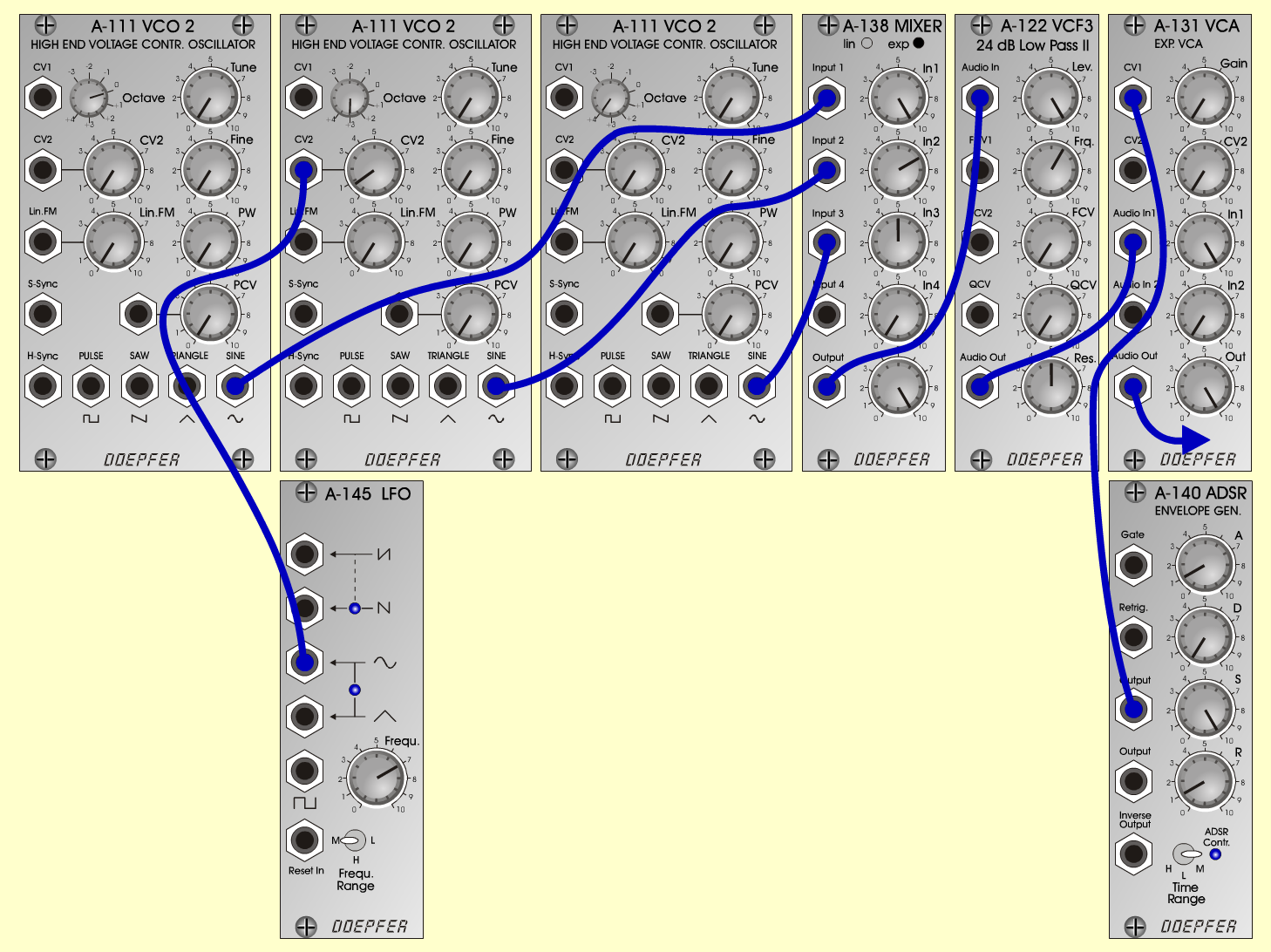E-OrganKeyboardsD o e p f e r A - 100 Connections: Settings: AA-111/1 (Sine)  <=>  A-138exp (Input 1) A-111/2 (Sine)  <=>  A-138exp (Input 2) A-111/3 (Sine)  <=>  A-138exp (Input 2) A-145 (Sine)  <=>  A-111/2 (CV2) A-138exp (Output)  <=>  A-122 (Audio In) A-122 (Audio Out)  <=>  A-131 (Audio In) A-140 (Output)  <=>  A-131 (CV 1) A-111/1 (Octave = 0) A-111/2 (CV 2 = 0.8, Octave = 3) A-111/3 (Octave = 4) A-138exp (In 1 = 10, In 2 = 7, In 3 = 5, Out = 10) A-122 (Level = 10, Freq = 6, Res = 5) A-145 (Frq = 7, Range = M) A-140 (A = 1, D = 0, S = 10, R = 1, Range = M) A-131 (Gain = 0, Audio In 1 = 10,  Audio Out = 10) Josef MuellerSound samples E-Organ-Piece E-Organ (261 Hz) E-Organ (523 Hz)